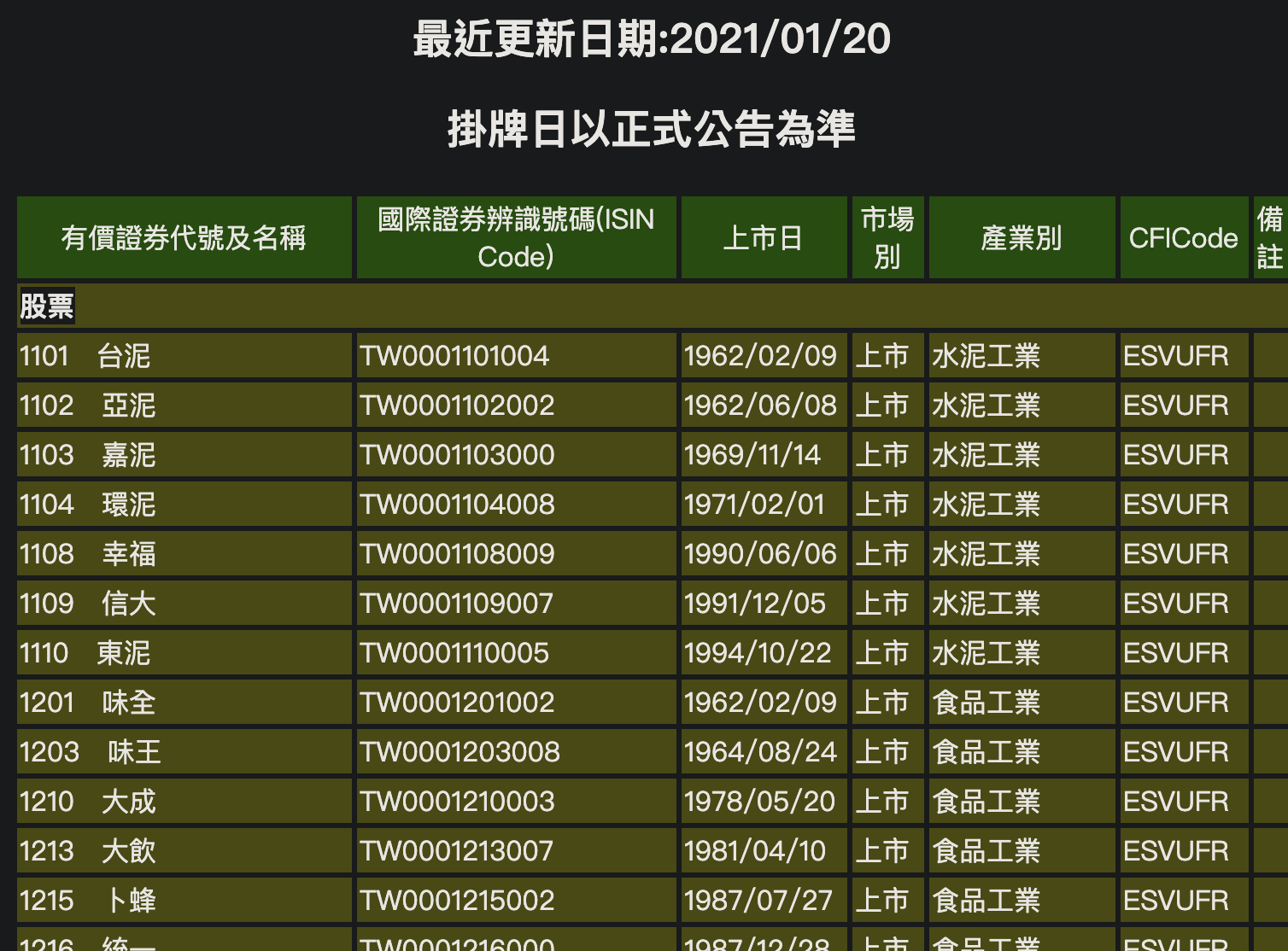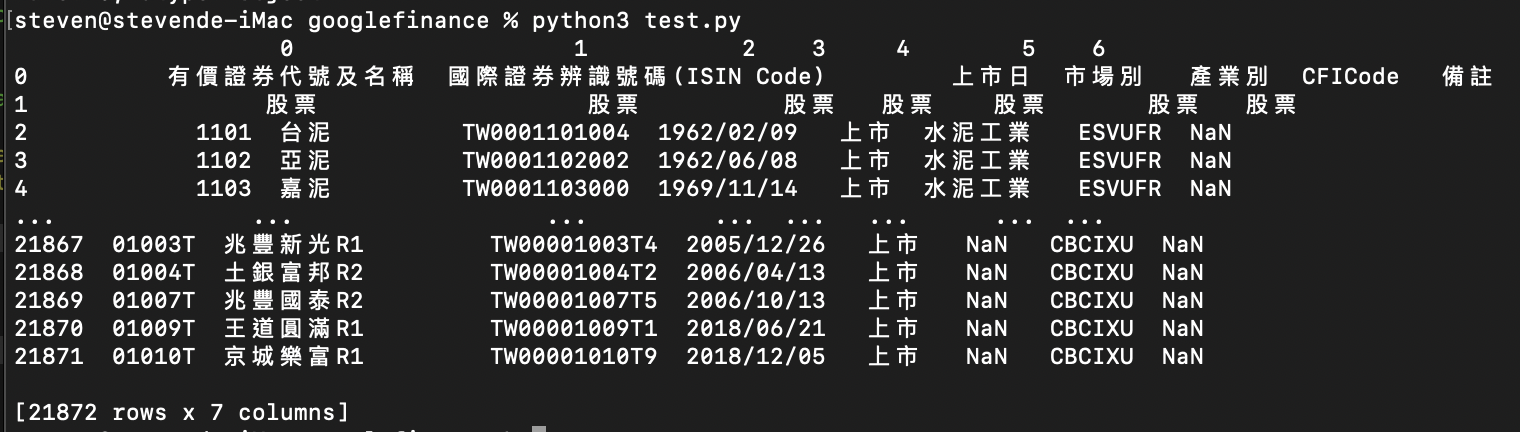# Python股票代碼抓取 上市股票一把抓

Python怎麼抓上市上櫃所有股票代碼?

• 文章目錄

## 1. 找尋所有股票代碼網站

http://isin.twse.com.tw/isin/C_public.jsp?strMode=2## 2. python解析html表格

<table style="width:100%">
<tr>
<th>Firstname</th>
<th>Lastname</th>
<th>Age</th>
</tr>
</table>


### 開始解析html

import requests
import pandas as pd

res = requests.get("http://isin.twse.com.tw/isin/C_public.jsp?strMode=2")
print(df)


### 結果## 3. 有價證券代號塞入欄位

['有價證券代號及名稱', '國際證券辨識號碼(ISIN Code)', '上市日', '市場別', '產業別', 'CFICode', '備註']


df.columns = ['有價證券代號及名稱', '國際證券辨識號碼(ISIN Code)', '上市日', '市場別', '產業別', 'CFICode', '備註']


## 4. 過濾股票代碼

### python正規表達式

import re
pattern = "[0-9]"
if re.search(pattern, 文字):
# 符合純數字


### python字串切割

string = "1101　台泥"
splitList = string.split('　')
# splitList = 1101
# splitList = 台泥


## 5. 最終程式碼

import requests
import pandas as pd
import re

res = requests.get("http://isin.twse.com.tw/isin/C_public.jsp?strMode=2")
df.columns = ['有價證券代號及名稱', '國際證券辨識號碼(ISIN Code)', '上市日', '市場別', '產業別', 'CFICode', '備註']

stackCodeDict = {}
for code in df['有價證券代號及名稱']:
pattern = "[0-9]"
stackCode = code.split('　')
if stackCode and re.search(pattern, stackCode) and len(stackCode) == 4:
print(str(stackCode) + stackCode)
stackCodeDict[stackCode] = stackCode

print(stackCodeDict)



## 6. 輸出結果Python更多文章

[教學] Line Bot 機器人不求人 – 範例 (Python)

Python – Selenium 繞過”這個瀏覽器或應用程式可能有安全疑慮”

python 各種均線 10MA/20MA均線 | pandas安裝

python sleep 暫停幾秒執行 – 2021年

PyAutoGUI Python控制電腦 | 按鍵精靈 | 鍵盤控制

python binance币安利用ccxt完成虛擬貨幣自動化交易

python write dictionary to text file | dict 寫入讀取

Categorized in: